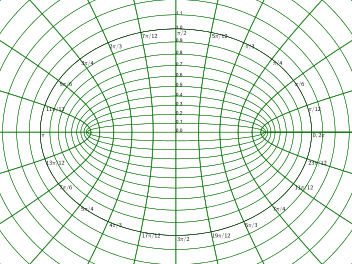# Elliptic coordinate system

Not to be confused with Ecliptic coordinate system.Elliptic coordinate system

In geometry, the elliptic(al) coordinate system is a two-dimensional orthogonal coordinate system in which the coordinate lines are confocal ellipses and hyperbolae. The two fociandare generally taken to be fixed atand, respectively, on the-axis of the Cartesian coordinate system.

## Basic definition

The most common definition of elliptic coordinatesiswhereis a nonnegative real number andOn the complex plane, an equivalent relationship isThese definitions correspond to ellipses and hyperbolae. The trigonometric identityshows that curves of constantform ellipses, whereas the hyperbolic trigonometric identityshows that curves of constantform hyperbolae.

## Scale factors

In an orthogonal coordinate system the lengths of the basis vectors are known as scale factors. The scale factors for the elliptic coordinatesare equal toUsing the double argument identities for hyperbolic functions and trigonometric functions, the scale factors can be equivalently expressed asConsequently, an infinitesimal element of area equalsOther differential operators such asandcan be expressed in the coordinatesby substituting the scale factors into the general formulae found in orthogonal coordinates.

## Alternative definition

An alternative and geometrically intuitive set of elliptic coordinatesare sometimes used, whereand. Hence, the curves of constantare ellipses, whereas the curves of constantare hyperbolae. The coordinatemust belong to the interval [-1, 1], whereas thecoordinate must be greater than or equal to one.

The coordinateshave a simple relation to the distances to the fociand. For any point in the plane, the sumof its distances to the foci equals, whereas their differenceequals. Thus, the distance tois, whereas the distance tois. (Recall thatandare located atand, respectively.)

A drawback of these coordinates is that the points with Cartesian coordinates (x,y) and (x,-y) have the same coordinates, so the conversion to Cartesian coordinates is not a function, but a multifunction.## Alternative scale factors

The scale factors for the alternative elliptic coordinatesareHence, the infinitesimal area element becomesand the Laplacian equalsOther differential operators such asandcan be expressed in the coordinatesby substituting the scale factors into the general formulae found in orthogonal coordinates.

## Extrapolation to higher dimensions

Elliptic coordinates form the basis for several sets of three-dimensional orthogonal coordinates. The elliptic cylindrical coordinates are produced by projecting in the-direction. The prolate spheroidal coordinates are produced by rotating the elliptic coordinates about the-axis, i.e., the axis connecting the foci, whereas the oblate spheroidal coordinates are produced by rotating the elliptic coordinates about the-axis, i.e., the axis separating the foci.

## Applications

The classic applications of elliptic coordinates are in solving partial differential equations, e.g., Laplace's equation or the Helmholtz equation, for which elliptic coordinates are a natural description of a system thus allowing a separation of variables in the partial differential equations. Some traditional examples are solving systems such as electrons orbiting a molecule or planetary orbits that have an elliptical shape.

The geometric properties of elliptic coordinates can also be useful. A typical example might involve an integration over all pairs of vectorsandthat sum to a fixed vector, where the integrand was a function of the vector lengthsand. (In such a case, one would positionbetween the two foci and aligned with the-axis, i.e.,.) For concreteness,,andcould represent the momenta of a particle and its decomposition products, respectively, and the integrand might involve the kinetic energies of the products (which are proportional to the squared lengths of the momenta).